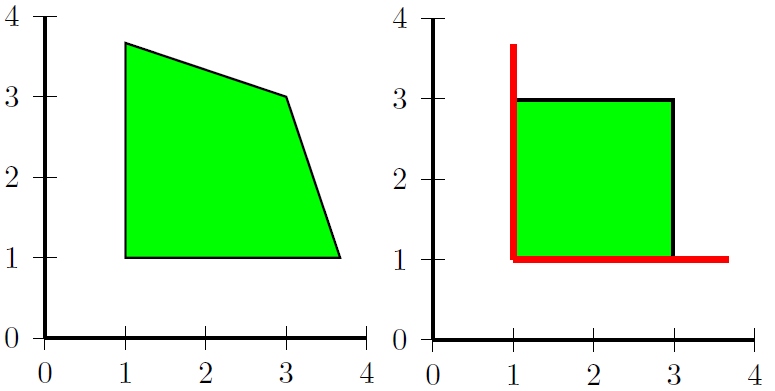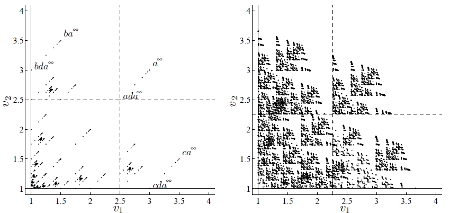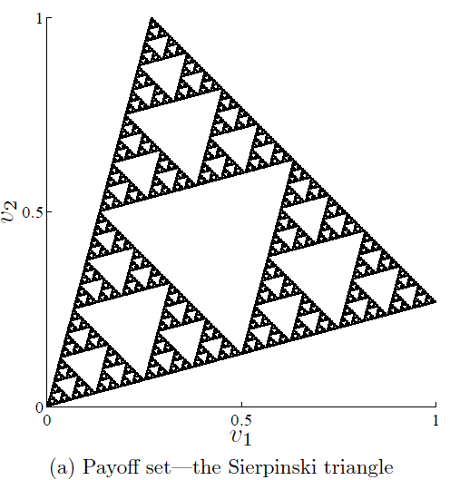# Kimmo Berg

## Repeated Games

The players' behavior changes when they play the same game for multiple periods. Take for example a game called Prisoner's Dilemma (Flood, Dresher and Tucker in 1950s, the payoffs are originally from Aumann 1981):

 Cooperate Defect Cooperate 3,3 0,4 Defect 4,0 1,1

When the game is played once, the solution (Nash equilibrium 1950) is (Defect,Defect) and both players get payoff 1. But when the game is repeated, it is possible to sustain a better outcome (Cooperate,Cooperate) where they both get payoff 3. How can the players sustain this solution when they both have short-term incentives to deviate to Defect with payoff 4 and what other solutions are there?

The equilibrium behavior and the fact that the players do not deviate from the equilibrium are based on threats what will happen if they deviate. In subgame-perfect equilibrium (Selten 1965) the threats need to be credible, i.e., the players cannot threaten with something that they are not willing to do when it is time to actually punish for the deviation. In Prisoner's dilemma, the optimal punishment is to play Defect and the other player can only guarantee payoff 1 by playing Defect as well. When the game is played many times, the player may then think whether it is better to deviate and get the payoff sequence (4,1,1,1,1...) or stick with the cooperation and get (3,3,3,...). Which one is better depends on the players preferences.

But playing cooperation all the time is not the only outcome when the players are patient enough. Folk theorem (Friedman 1971) tells that any solution that gives strictly higher payoff than the punishment value to all players is a solution to the game, which means that there is a huge number of solutions (see the green area in left pic below). In discounted games where the players enjoy today's payoffs more than the future payoffs, this was proven in infinitely repteated games by Fudenberg and Maskin (1986).But what if the player are not arbitrarily patient but value current payoffs dramatically more than the payoffs long in the future? The patience can be described by a discount factor d and the players use geometric discounting to evaluate the sequence of payoffs (4,1,1,1...) as 4+d*1+d^2*1+d^3*1+... When d is close enough to 1, the players prefer sequence (3,3,3,...) but when d is close to zero (which corresponds to playing the game only once) the only solution is to defect. In Berg and Kitti "Equilibrium Paths in Discounted Supergames" we describe how all pure-strategy solutions (including both paths and payoffs) can be found when the players' discount factors are given. The figure below illustrates the possible payoffs for two different discount factors: d=0.5 and d=0.58. We can see that the number of solutions increase when the players become more patient.In Berg and Schoenmakers "Construction of randomized subgame-perfect equilibria in repeated games" we extend the model to randomized strategies where the players can only observe the realized pure actions (not the probabilities that the other players are using). We extend the Abreu-Pierce-Stacchetti (1986,1990) fixed-point characterization and observe that some payoffs can be obtained already with very low discount factor values and it is very difficult to find all solutions. We also show some results for the Prisoner's Dilemma, see the green and red areas in right pic above for d=1/3.

The algorithm for computing pure-strategy subgame-perfect solutions is described in Berg and Kitti (2013) and in Berg and Kitti (2014) we classify the solution sets as fractals called self-affine sets. It is possible to evaluate how dense the solution set is by estimating the eigenvalue of the graph that generates all the equilibrium paths or by estimating the Hausdorff dimension of the payoff set. These measures make it possible to compare the solution sets between games and analyze how the sets enlarge when the players become more patient. We have also found a game called Sierpinski game, whose solution set is the famous Sierpisnki triangle given below.